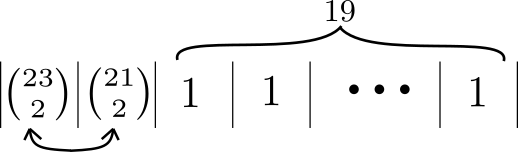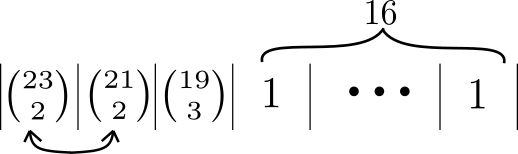Posted on 05 June, 2021

Exact probabilities for counting the chance of collisions with distinct objects, using the birthday problem as a working example.

Introduction

This follows from my previous blog post on Intuitive explanations for non-intuitive problems: the Birthday Problem. Please see that post for a heuristic understanding of the problem. It assumed independence of sharing birthdays. This post makes no such assumption, so the mathematics is more advanced. A background in counting methods with combinations and permutations is required.

I have divided this section in to the following parts:

Probability of $k$ collisions

What is the chance of $i$ people in a group of $k$ people sharing a birthday? In the previous post I assumed independence between sharing birthdays. But sharing is dependent. If person A and person B share a birthday, then sharing a birthday with person B depends on sharing a birthday with person A. Counting methods can account for this dependence.

In a group of 23 people there are ${23 \choose 2}=253$ ways that two people can be chosen. Separately we can imagine filling 22 slots with distinct birthdays (not 23 because one is shared). There are $^{365}P_{22}$ ways of filling these slots. The total probability is then:

\begin{align} P(x=2 | k=23) &= {23 \choose 2} \frac{^{365}P_{22}}{365^{23}} \\ &= 0.3634 \end{align}

Similarly for three people sharing a birthday:

\begin{align} P(x=3 | k=23) &= {23 \choose 3} \frac{^{365}P_{21}}{365^{23}} \\ &= 0.0074 \end{align}

This is much more unlikely.

Probability of groups of collisions

There can also be two pairs of two people sharing birthdays. Or three pairs. Or two pairs and a triplet and a quintuplet.

First let’s take the case of two pairs of two people amongst 23 people in total. We first choose 2 to be part of the first pair ($\binom{23}{2}$), then 2 of the remaining 21 to be part of the second pair ($\binom{21}{2}$). We can shuffle the birthdays between 21 unique slots ($^{365}P_{21}$). The first two slots can be shuffled without changing the arrangement because they both have identical looking pairs ($2!$). The probability is then:\begin{align} P(x=(2,2) | k=23) &= \frac{\binom{23}{2}\binom{21}{2}}{2!}\frac{^{365}P_{21}}{365^{23} } \\ &= \frac{23!}{(2!)^2 2!19!} \frac{^{365}P_{21}}{365^{23}} \\ &= 0.1109 \end{align}

Now let’s add a set of three:\begin{align} P(x=(2,2,3) | k=23) &= \frac{\binom{23}{2}\binom{21}{2}}{2!}\binom{19}{3} \frac{^{365}P_{19}}{365^{23} } \\ &= \frac{23!}{(2!)^2 2!3!16!} \frac{^{365}P_{19}}{365^{23}} \\ &= 0.0009 \end{align}

This is much more unlikely.

To get the probability of at least 2 people sharing, we need to account for all these scenarios. We can go through all ways of partitioning $k$ people. This is known as the integer partition problem. In practice, I find it is accurate enough to only consider up to 3 or 4 people sharing a birthday, because the other scenarios are so unlikely. However the method in the next section is much simpler.

Probability of at least 1 collision

As in my previous post, it’s easiest to work backwards. First find the probability that no people share a birthday and then subtract this from 1. For $k$ people, we can imagine shuffling $k$ unique birthdays in $k$ slots:

\begin{align} P(x \geq 1 | k) &= 1 - \frac{^{365}P_{k}}{365^k} \end{align}

For 23 people this gives a probability of 0.5073. The previous sections show that of the collisions that happen, 73% will be just two people, and 22% two pairs of two people.

Probability of collisions when drawing from a distribution

A rather subtle but important assumption made in the previous sections is that we are drawing people from a large population. This assumption breaks down when drawing from a small sample. For example, Facebook friends. We can only draw as many pairs, triples and sets in general as exist. In my group of friends there is a day where seven people share a birthday but none where six do. So I cannot include this probability in my calculation, which the calculation in the previous section does.

The probability of drawing from a distribution is a tricky problem. One notable application is calculating probabilities of hands in poker.

It is simpler and quicker to run Monte Carlo simulations, as I did in my previous post. Here is a comparison of running 1,000 and 10,000 trials per point compared to the true curve:

The Monte Carlo simulations hold up well. The MC 10,000 curve is almost indistinguishable from the theoretical curve.

But how does one calculate the theoretical curve? Let’s start with a simpler, very specific problem. What if I want to select 30 friends of which none share birthdays, but 12 share birthdays with one other friend (not in the 30), and 2 share with two other friends each, and 1 is one of the 7 that share a birthday on the same day? (This is the simpler problem.)

• There are $\binom{374}{30}$ ways in total to choose 30 people.
• From the $n_2=73$ pairs, I want to select 10 pairs $\binom{73}{10}$, 1 of 2 from each pair $\binom{2}{1}^{10}$.
• From the $n_3=13$ triples, I want to select 2 triples $\binom{13}{2}$, 1 of 3 from each triple $\binom{3}{1}^{2}$.
• From the $n_7=1$ sevens, I want to select 1 person of 7 $\binom{7}{1}$.
• The remaining $17$ all come from the set of $n_1=132$ singeltons $\binom{132}{17}$.

Hence the total probability is: \begin{align} P&(x =(1\times 17, 2\times 10, 3\times 2, 7\times 1)|k=30) \\ &= \left[ \binom{73}{10} \binom{2}{1}^{10} \right] \left[ \binom{13}{2} \binom{3}{1}^2 \right] \left[ \binom{1}{1} \binom{7}{1} \right] \binom{132}{17} \div \binom{374}{30} \\ &= 0.00001981 \end{align}

Now repeat this calculation for every possible way of making up 30 friends from the known distribution. This can be done with an integer partition algorithm with a maximum on the values that each partition can take. This will give the theoretical value of selecting zero friends who share birthdays. Subtract from 1 to get the probability of least 2 sharing a birthday.

For Julia code on my laptop, this takes 22.5 seconds. Monte Carlo simulations with 10,000 trials per each point from 1 to 60 takes 2.2 seconds for all 600,000 trials. So the Monte Carlo simulations are faster.

If there is an easier way please let me know 🙂.

Integer partitions

For completeness, here is code for the integer partition problem implemented in Julia.

If $p(n)$ is an integer partition of $n$, then $p(n) = k + p(n -k)$. The following recursive algorithm is based off this formula:

This function gives all the unique partitions of a number. For the above problems we have the additional constraints:

• There is a maximum value at each partition index.
• Permutations of partitions are unique e.g. 3+0+0, 0+3+0 and 0+0+3 are all unique.

The function is therefore modified to keep an index count:

Caching can be added to improve the performance.